TitleIntermediate Algebra
Answer/Discussion to Practice Problems
Tutorial 31:Practice Test on Tutorials 23 - 30

 Problem 1a: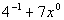Answer: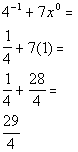Problem 1b:Answer: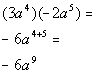Problem 1c: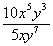Answer: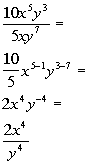Problem 1d: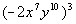Answer: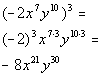Problem 1e: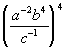Answer: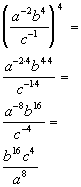Problem 1f: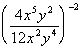Answer: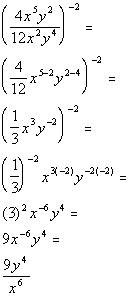Problem 2a:

 73,200,000Answer: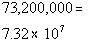Problem 3a: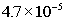Answer: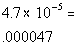Problem 4a: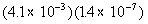Answer: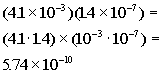Problem 5a: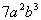Answer: Since the degree is the sum of the variable exponents and it looks like we have a 2 and a 3 as our exponents, the degree would have to be 2 + 3 = 5.

 Problem 5b:

 -8Answer: Since this is a constant term, it's degree is 0.

 Problem 6a: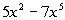Answer: Since the degree of the polynomial is the highest degree of all the terms, it looks like the degree is 5. Since there are two terms, this is a binomial.

 Problem 6b: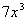Answer: Since the degree of the polynomial is the highest degree of all the terms, it looks like the degree is 3. Since there is one term, this is a monomial.

 Problem 6c: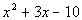Answer: Since the degree of the polynomial is the highest degree of all the terms, it looks like the degree is 2. Since there are two terms, this is a trinomial.

 Problem 7a: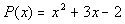;  P(-2)Answer: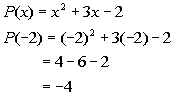Problem 8a: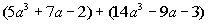Answer: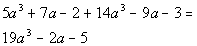Problem 8b: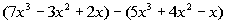Answer: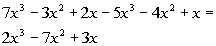Problem 9a: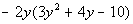Answer: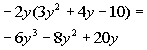Problem 9b: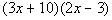Answer: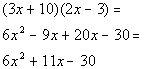Problem 9c: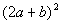Answer: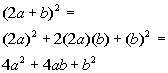Problem 9d: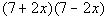Answer: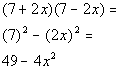Problem 9e: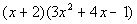Answer: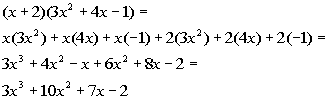Problem 10a: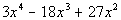Answer: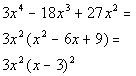Problem 10b: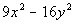Answer: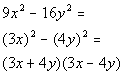Problem 10c: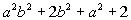Answer: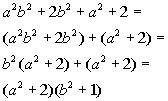Problem 10d: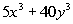Answer: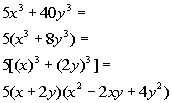Problem 10e: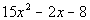Answer: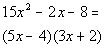Problem 11a: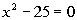Answer: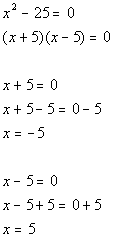x = -5, 5

 Problem 11b: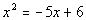Answer: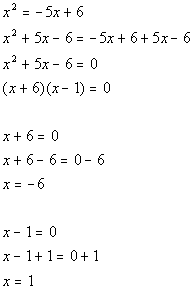x = -6 and 1

 Problem 11c: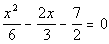Answer: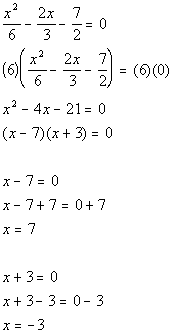x = 7, -3

Last revised on July 16, 2011 by Kim Seward.
All contents copyright (C) 2001 - 2011, WTAMU and Kim Seward. All rights reserved.